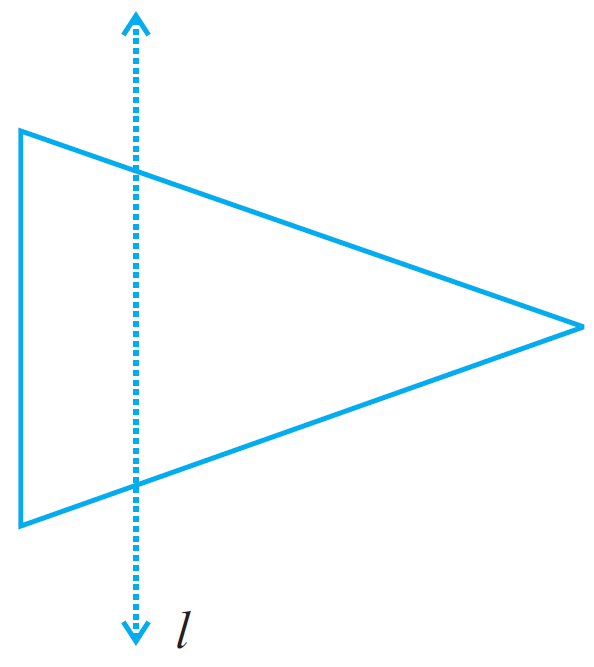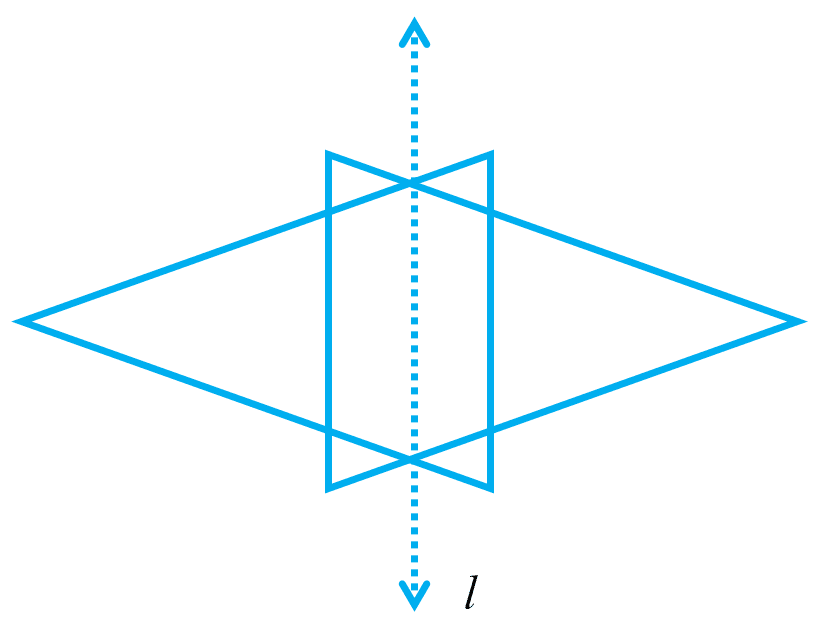# vs.eyeandcontacts.com

## Chapter 13 Symmetry Exercise 13.1

Question 1: List any four symmetrical objects from your home or school.
Chocolate, Table, Board, Clock etc.

Question 2: For the given figure, which one is the mirror line, l₁ or l₂?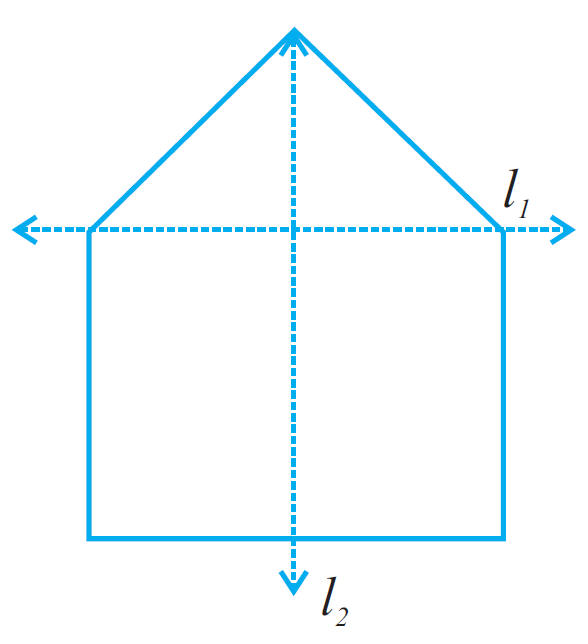Answer: l₂ is the mirror line because it divides the figure into two equal half.

Question 3: Identify the shapes given below. Check whether they are symmetric or not. Draw the line of symmetry as well.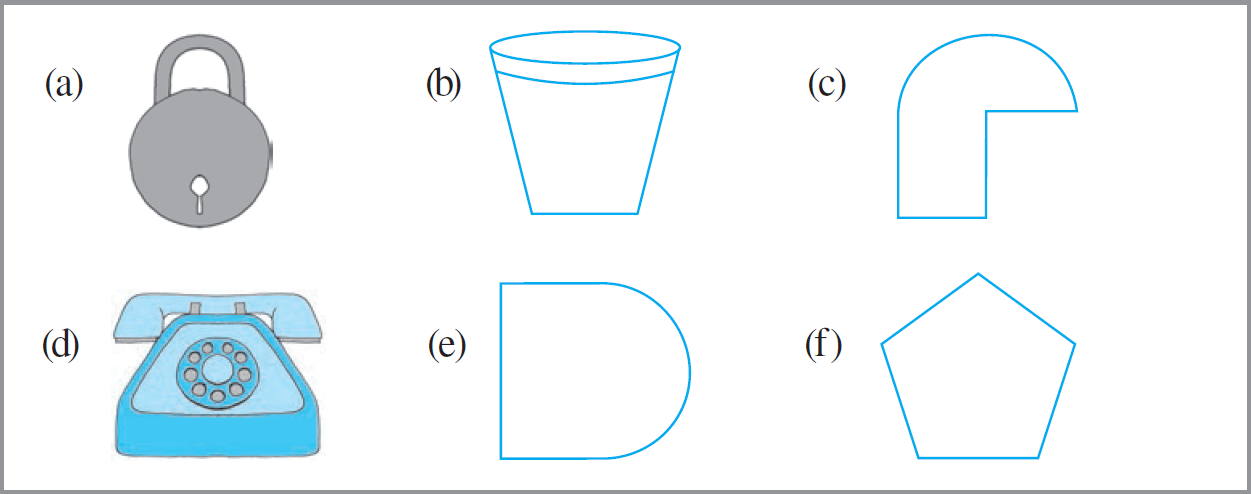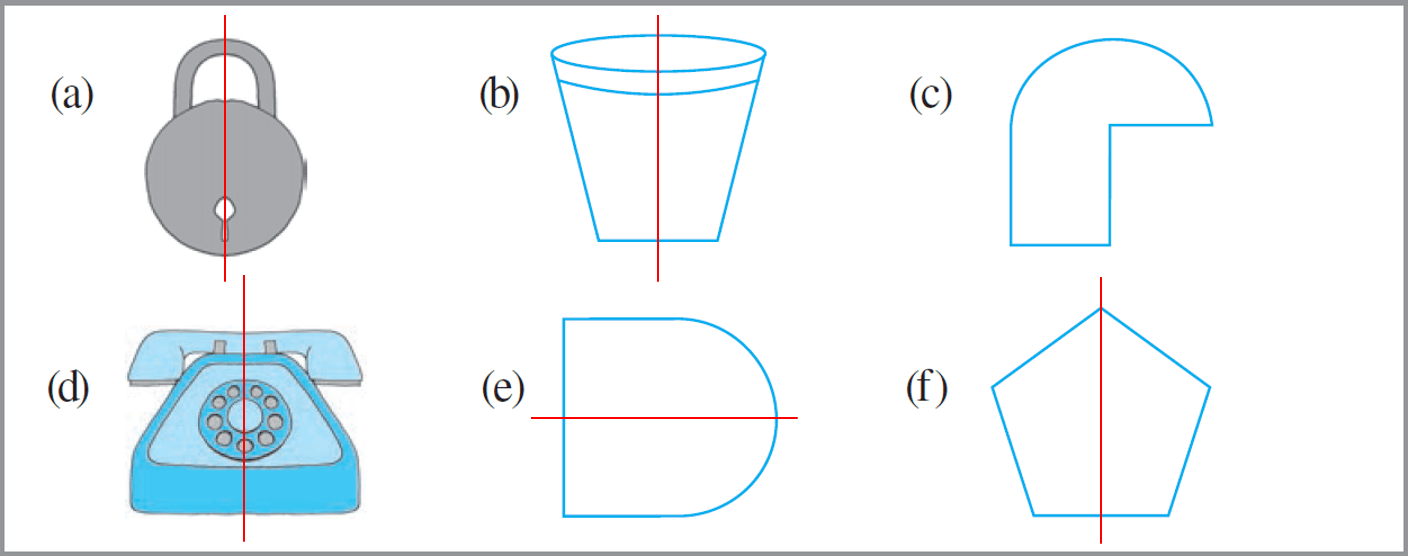a) Yes it is symmetric.
b) Yes it is symmetric.
c) No it is symmetric.
d) Yes it is symmetric.
e) Yes it is symmetric.
f) Yes it is symmetric.

Question 4: Copy the following on a squared paper. A square paper is what you would have used in your arithmetic notebook in earlier classes. Then complete them such that the dotted line is the line of symmetry.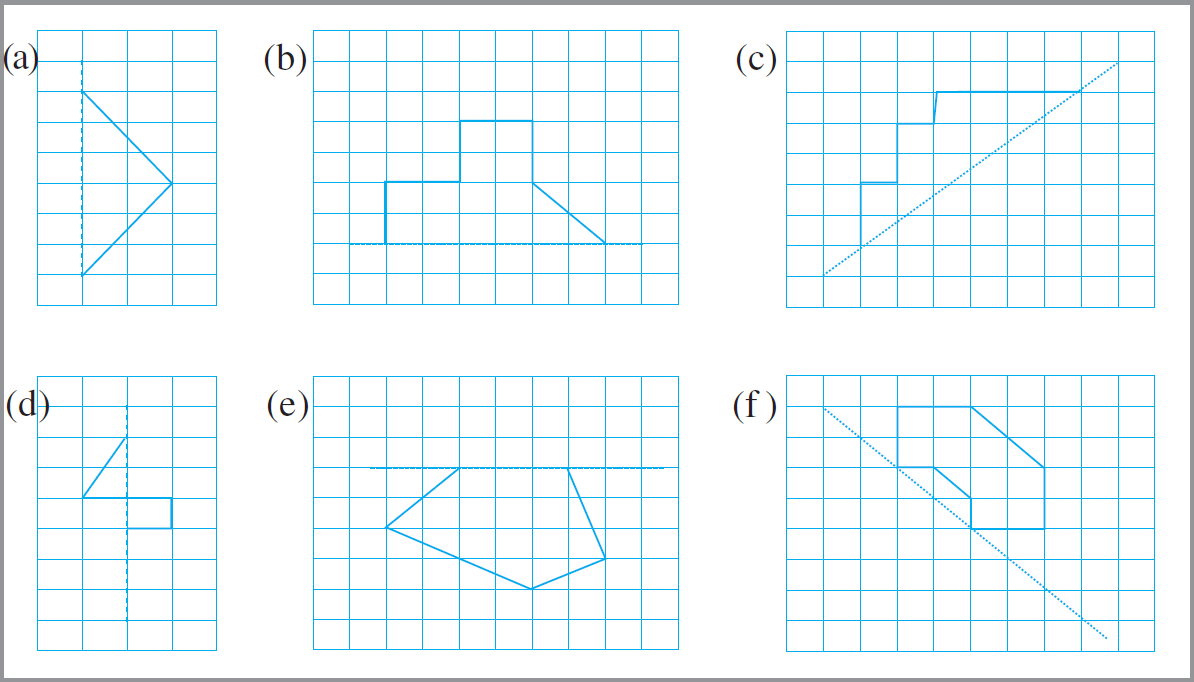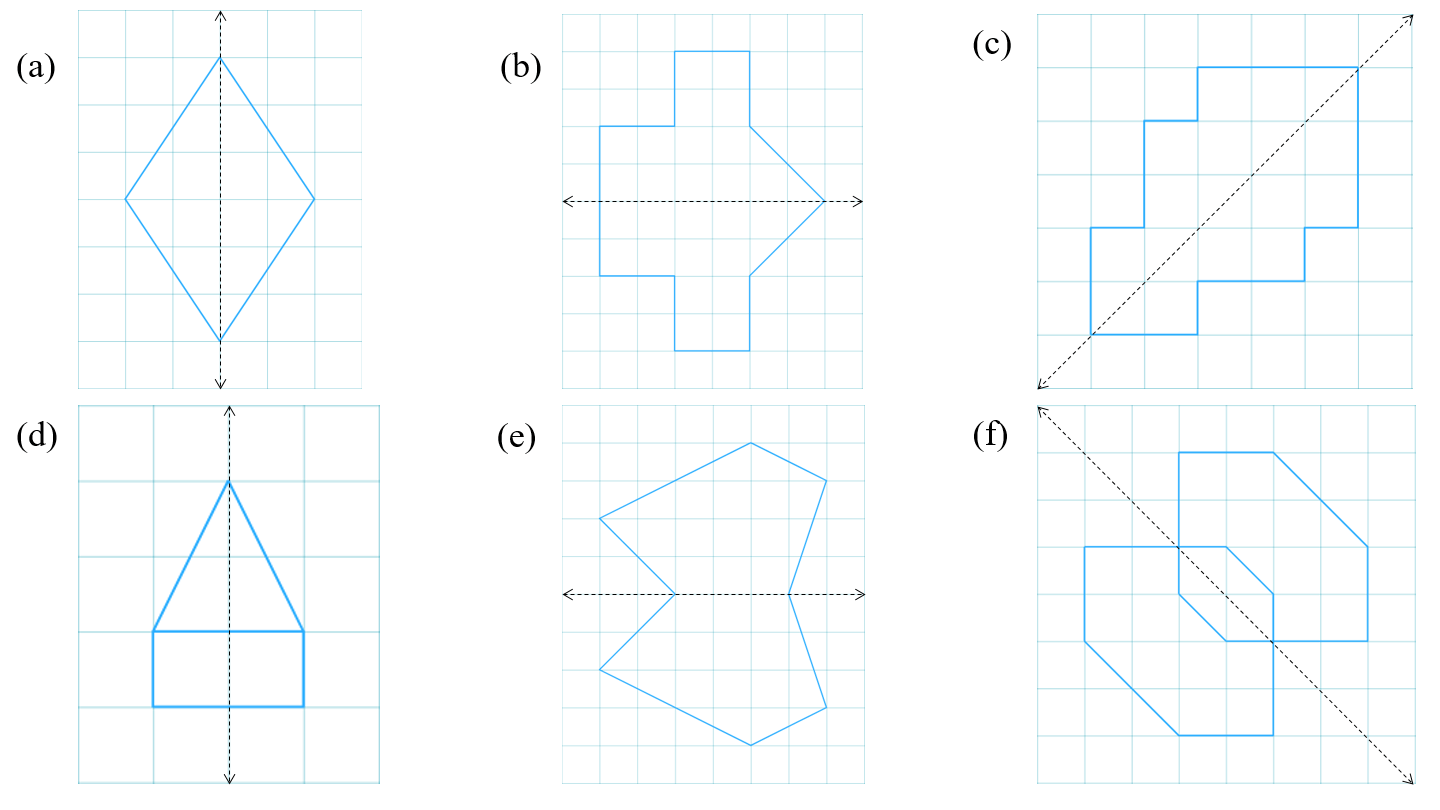Question 5: In the figure, l is the line of symmetry. Complete the diagram to make it symmetric.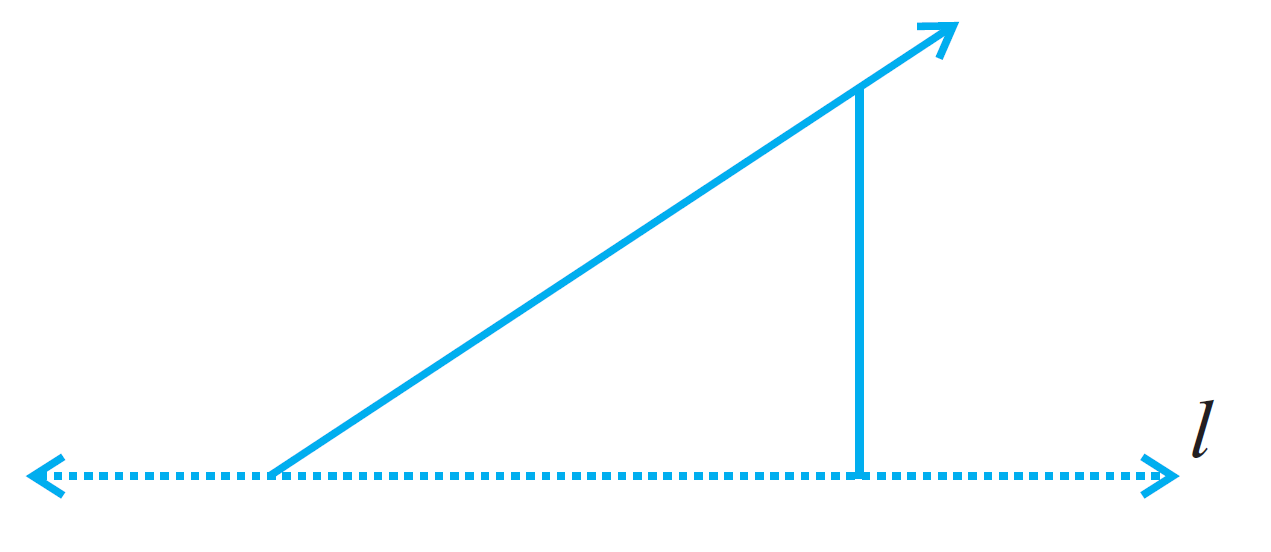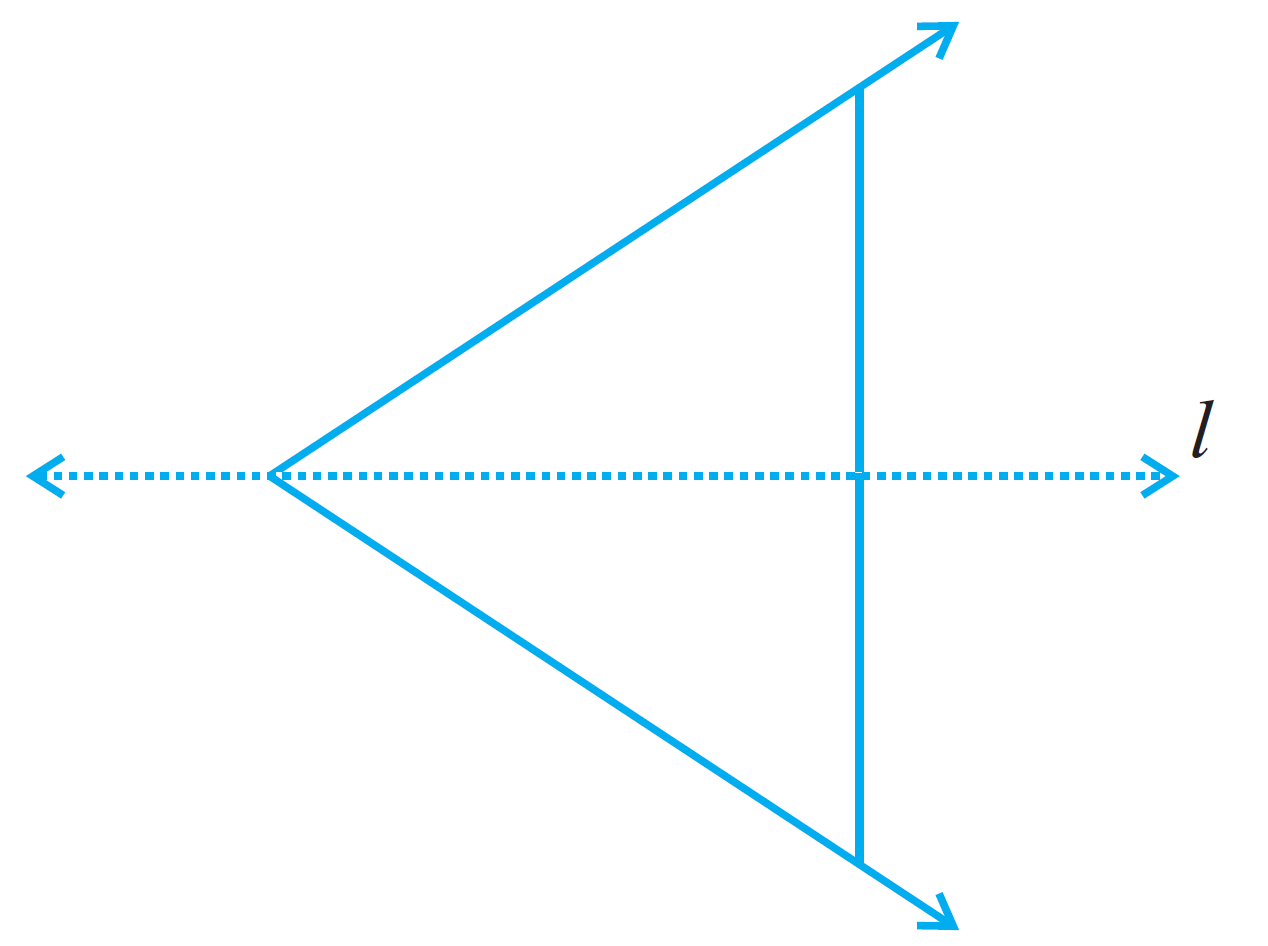Question 6: In the figure, l is the line of symmetry. Draw the image of the triangle and complete the diagram so that it becomes symmetric.